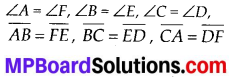## MP Board Class 7th Maths Solutions Chapter 7 Congruence of Triangles Ex 7.1

Question 1.
Complete the following statements:
(a) Two line segments are congruent if ___ .
(b) Among two congruent angles, one has a measure of 70°; the measure of the other angle is ___.
(c) When we write ∠A = ∠B, we actually mean ___.
Solution:
(a) They have the same length.
(b) 70°
(c) m∠A = m∠BQuestion 2.
Give any two real-life examples for congruent shapes.
Solution:
(i) Sheets of same letter pad.
(ii) Biscuits in the same packet.

Question 3.
If ∆ABC ≅ ∆FED under the correspondence ABC ⟷ FED, write all the corresponding congruent parts of the triangles.
Solution:
If ∆ABC ≅ ∆FED, then the corresponding angles and sides will be equal to each other.Question 4.
If ∆DEF ⟷ ∆BCA, write the part(s) of ∆BCA that correspond to
(i) ∠E
(ii) $$\overline{E F}$$
(iii) ∠F
(iv) $$\overline{D F}$$
Solution:
(i) ∠C
(ii) $$\overline{C A}$$
(iii) ∠A
(iv) $$\overline{B A}$$